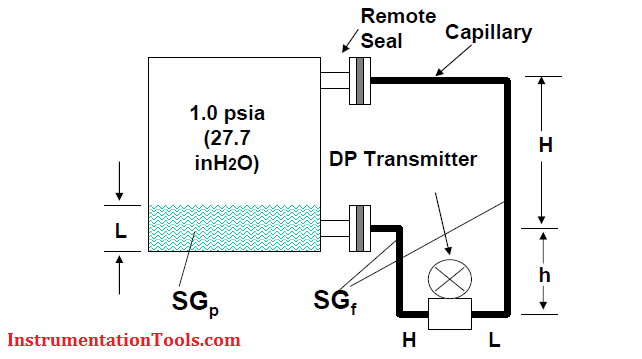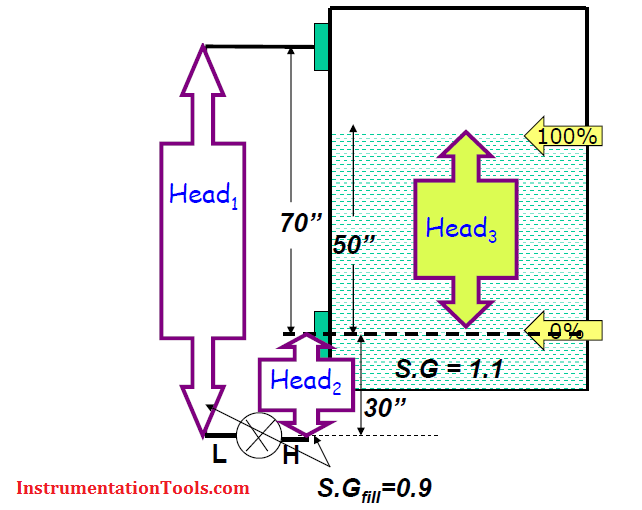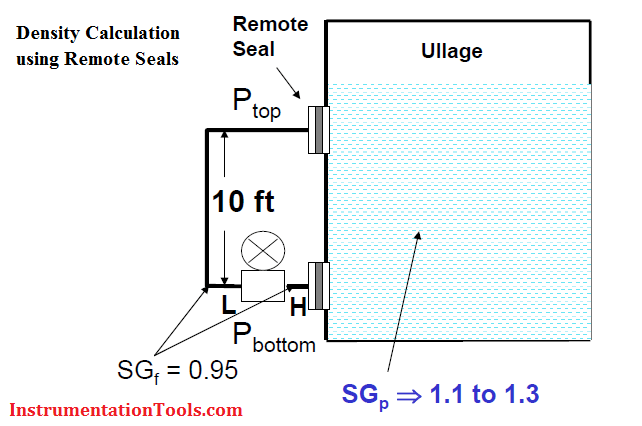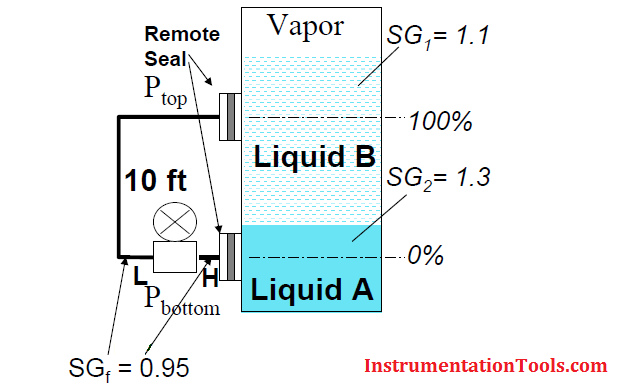Home » Level Calculation with Remote Seals

Level Calculation with Remote Seals

Diaphragm seals for tanks under Vacuum

The transmitter must be mounted level with or below the lowest tap to ensure positive pressure at the transmitter.Double Remote Seal ApplicationCalibration Range

LRV or 4mA point = Phigh – Plow

LRV = (0.9*30”) – (0.9*100”)

LRV = -63”H2O

URV or 20mA Point = Phigh – Plow

URV = [(0.9*30”) + (1.1*50”)] – (0.9*100”)

URV = -8”H2O

Cal. Range = -63 to -8 in H2O

Density Calculation with Remote Seals TransmitterMax. Allowable ΔS.G = 0.2

Dist. Betw. Taps = 10 ft = 10 x 12” = 120”

Calibration Range

LRV or 4mA point = 120” * (SGp – SGf)

LRV = 120” * (1.1 – 0.95)

LRV = 18”H2O

URV or 20mA Point = 120” * (SGp – SGf)

URV = 120” * (1.3 – 0.95)

URV = 42”H2O

Cal. Range = 18 to 42 inH2O

Interface Calculation with Remote Seals Transmitter

Application Example: To determine % of interface of Liquid A with respect to Liquid B.Dist. Betw. Taps = 10 ft = 10 x 12” = 120”

Calibration Range

LRV or 4mA point = 120” * (SG1 – SGf)

LRV = 120” * (1.1 – 0.95)

LRV = 18”H2O

URV or 20mA Point = 120” * (SG2 – SGf)

URV = 120” * (1.3 – 0.95)

URV = 42”H2O

Cal. Range = 18 to 42 inH2O

Calculate Flow Transmitter Output CurrentMay 6, 2016 at 1:38 am

Thanks for sharing the Remote Seals Level Calibration ProcedureJuly 27, 2016 at 8:46 am

wow this is fantastic

This website uses cookies to improve your experience. We'll assume you're ok with this, but you can opt-out if you wish. Accept Read More

WordPress Image Lightbox

Send this to a friend